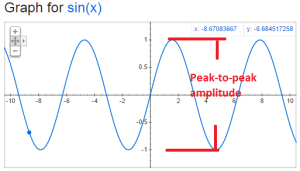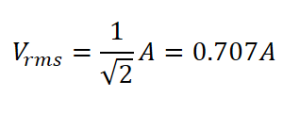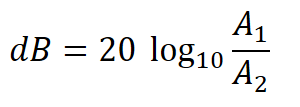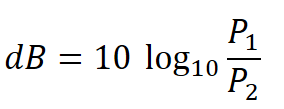# 1.08 Signal amplitudes and decibels

Peak-to-peak amplitude (pp amplitude) is twice the amplitude. This is the measurement of the amplitude from the bottom peak to the top peak as illustrated below:root-mean-square amplitude (rms amplitude) has different formulas based upon the shape of the signal.  For sine waves only it is given by:Decibels is a comparison between two signals.

When comparing amplitude use this formula:When comparing the power use this formula:Sometimes decibels are presented as units.  This is because their is an implicit signal that is being used for comparison.  Here are some common ones:

a) dBV:  1 volt rms

b) dBm – the voltage corresponding to 1mW with an assumed load impedance

c) small noise generated by a resistor at room temperature